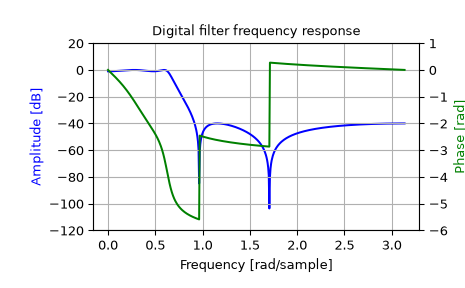# scipy.signal.iirdesign¶

scipy.signal.iirdesign(wp, ws, gpass, gstop, analog=False, ftype='ellip', output='ba', fs=None)[source]

Complete IIR digital and analog filter design.

Given passband and stopband frequencies and gains, construct an analog or digital IIR filter of minimum order for a given basic type. Return the output in numerator, denominator (‘ba’), pole-zero (‘zpk’) or second order sections (‘sos’) form.

Parameters
wp, wsfloat or array like, shape (2,)

Passband and stopband edge frequencies. Possible values are scalars (for lowpass and highpass filters) or ranges (for bandpass and bandstop filters). For digital filters, these are in the same units as fs. By default, fs is 2 half-cycles/sample, so these are normalized from 0 to 1, where 1 is the Nyquist frequency. For example:

• Lowpass: wp = 0.2, ws = 0.3

• Highpass: wp = 0.3, ws = 0.2

• Bandpass: wp = [0.2, 0.5], ws = [0.1, 0.6]

• Bandstop: wp = [0.1, 0.6], ws = [0.2, 0.5]

For analog filters, wp and ws are angular frequencies (e.g., rad/s). Note, that for bandpass and bandstop filters passband must lie strictly inside stopband or vice versa.

gpassfloat

The maximum loss in the passband (dB).

gstopfloat

The minimum attenuation in the stopband (dB).

analogbool, optional

When True, return an analog filter, otherwise a digital filter is returned.

ftypestr, optional

The type of IIR filter to design:

• Butterworth : ‘butter’

• Chebyshev I : ‘cheby1’

• Chebyshev II : ‘cheby2’

• Cauer/elliptic: ‘ellip’

• Bessel/Thomson: ‘bessel’

output{‘ba’, ‘zpk’, ‘sos’}, optional

Type of output: numerator/denominator (‘ba’), pole-zero (‘zpk’), or second-order sections (‘sos’). Default is ‘ba’ for backwards compatibility, but ‘sos’ should be used for general-purpose filtering.

fsfloat, optional

The sampling frequency of the digital system.

New in version 1.2.0.

Returns
b, andarray, ndarray

Numerator (b) and denominator (a) polynomials of the IIR filter. Only returned if output='ba'.

z, p, kndarray, ndarray, float

Zeros, poles, and system gain of the IIR filter transfer function. Only returned if output='zpk'.

sosndarray

Second-order sections representation of the IIR filter. Only returned if output=='sos'.

butter

Filter design using order and critical points

cheby1, cheby2, ellip, bessel
buttord

Find order and critical points from passband and stopband spec

cheb1ord, cheb2ord, ellipord
iirfilter

General filter design using order and critical frequencies

Notes

The 'sos' output parameter was added in 0.16.0.

Examples

>>> from scipy import signal
>>> import matplotlib.pyplot as plt
>>> import matplotlib.ticker

>>> wp = 0.2
>>> ws = 0.3
>>> gpass = 1
>>> gstop = 40

>>> system = signal.iirdesign(wp, ws, gpass, gstop)
>>> w, h = signal.freqz(*system)

>>> fig, ax1 = plt.subplots()
>>> ax1.set_title('Digital filter frequency response')
>>> ax1.plot(w, 20 * np.log10(abs(h)), 'b')
>>> ax1.set_ylabel('Amplitude [dB]', color='b')
>>> ax1.grid()
>>> ax1.set_ylim([-120, 20])
>>> ax2 = ax1.twinx()
>>> angles = np.unwrap(np.angle(h))
>>> ax2.plot(w, angles, 'g')
>>> ax2.grid()
>>> ax2.axis('tight')
>>> ax2.set_ylim([-6, 1])
>>> nticks = 8
>>> ax1.yaxis.set_major_locator(matplotlib.ticker.LinearLocator(nticks))
>>> ax2.yaxis.set_major_locator(matplotlib.ticker.LinearLocator(nticks))#### Previous topic

scipy.signal.group_delay

#### Next topic

scipy.signal.iirfilter